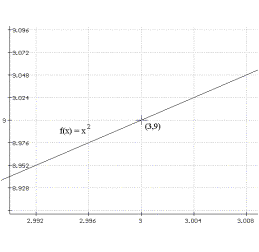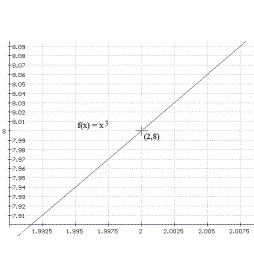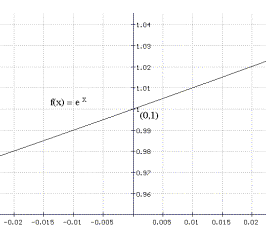Chapter I: Introduction to The Derivative
(Revised Draft Version 5-27-2014)

 How do we come to understand Mathematics:  The discussion of how we can best arrive at mathematical understanding has certainly been around for thousands of years. Current discussions of pedagogy (the art of instruction) focus attention on the learner developing understanding of concepts and techniques through a mixture of investigations, guided experiences, and problem solving. When possible we will try to build an understanding of calculus concepts on your prior physical and mathematical experiences and familiarity with aspects of common phenomena.

Preface: In this chapter we begin exploring one of the key concepts of the calculus, called the derivative. Though the definition of the derivative in a mathematical setting is primarily a matter of numbers and functions, the derivative is important for its use in various applications that employ the numerical and symbolic power of mathematics to study change. These applications have become important interpretations of the derivative concept, while providing powerful analytic tools for the construction of mathematical models. The interpretations  suggest and confirm the truth of mathematical results when the mathematics fits well with the experience of the interpretation. This symbiotic relation of mathematical abstractions with "real world" interpretations may be a reason for the unusual success mathematics has had in assisting scientific studies in so many disciplines. We start with some key motivation for the derivative concept.

In Section A we examine the geometric problem of describing lines that are tangent to a curve. This problem should be familiar to you already from Chapter 0.B3, though previously we considered it using only algebra.

Next we'll turn our attention in Section B to the physics problem of describing the velocity of an object moving along a straight line. These two problems have been key models in the historical development of the calculus.

In Section C, we'll consider models from probability and economics (also considered in Chapter 0) which in the 19 th and 20 th centuries have provided more applications for the calculus outside of the traditional physical and natural sciences. Further examples and applications will be found in the exercises illustrating the importance of  the study of change.With these motivating interpretations it should become apparent that there is a common mathematical concept used to analyze each of these models.
The mathematical abstraction of that common concept leads to the definition of the derivative in Section D. This concept has two key aspects, as a number and also as a function . The definition, its initial application to "finding the derivative," and other basic concepts in the study of the derivative will round out this chapter. Procedures for finding the derivative of function based primarily on the function's algebraic - symbolic character provide the first example of a calculus, a calculus for finding derivatives.
What lies beyond this chapter: More details of the calculus of derivatives will be covered in Chapter II. We'll return to an examination of some traditional applications of the derivative calculus in Chapter III. In Chapter IV we will reverse our investigations and examine how information about a derivative controls the possibilities for related "primitive" functions. Information about the derivative is usually presented in the form of derivative (or differential) equations. Trying to solve these equations provides a sensible transition to the second key concept of the calculus, the integral, which, like the derivative, is also both a number and a function. But for now we can put this second concept aside and proceed to discuss motivating interpretations for the derivative.
I. A. [Motivation] Finding the Slope of a Tangent Line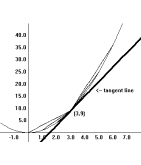The Tangent Problem Again: We are interested in finding the slope of a line that is tangent to a given curve at a specific point on that curve. In Chapter 0.B3 we considered the curve with equation $Y = X^2$ and  used the geometric characterization that a tangent line will meet the curve at only one point to investigate this problem. Algebra for the equations of the curve and the tangent line led us to conclude there was only one possible slope for the tangent line. our work showed that the slope depended only on the first coordinate of the point. In fact, for the point on the curve with coordinates (a,a2 ) we found that the slope of the tangent line had to be 2a. In this section we will follow a different approach to find the slope of the tangent line. Instead of finding the slope using algebra alone, we will begin by estimating the value of this slope, combining algebra with our common sense of what it means to be close.

 X Y Slope of Secant =$\frac {Y-9}{X-3}$ 0 0 3 1 1 4 2 4 5 4 16 7 5 25 8 6 36 9

Estimating (the slope of) the tangent line: Our estimates will depend on examining lines that are close to the tangent line. One difficulty in our analysis of the tangent problem is that we have not said precisely what a tangent line is. The word "close" is also imprecise. We will continue our investigations without resolving these issues here. The more experience one has investigating a concept, the more clear its meaning can become.  Articulation of a careful definition for "tangent" will make more sense later, based on greater experience .

Example I.A.1. Estimation and analysis for $Y = X^2$:
Let's reconsider the question treated in Chapter 0.B.3 o
f finding a lin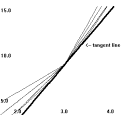e tangent to the graph of  $Y = X^2$ at the point (3,9). We call a line that passes through two (or more) distinct points on a curve a secant line of the curve. As Figure 1 illustrates, there are many secant lines to the graph of  $Y = X^2$ that pass through the point (3,9). Each of these secant lines is determined by the first coordinate of a point on the graph of  $Y = X^2$ other than (3,9). So using the first coordinates 0, 1, 2, 4, 5, and 6 we have different secant lines determined by the points (0,0), (1,1), (2,4), (4,16), (5,25), and (6,36). A glance again at Figure 1 should help in understanding that the lines determined by (2,4) and (4,16) are better approximations for the tangent line than the other lines in the figure.

Determining the slopes of secant lines provides a numerical approach  to finding the slope of the tangent line (previously discovered to be 6 in Example 0.B.***). In Table 1 we give these points and the slopes of the secant lines they determine with the point (3,9) using the formula $\frac {Y-9}{X-3}$. This table also helps in understanding that the slopes determined by using  (2,4) and (4,16) are better approximations for the slope of the tangent line than the other slopes in the table.

It seems likely from both the visual and numerical views that to obtain even better estimates of the tangent line and its slope we should investigate secant lines determined by points (x, x2) with the first coordinate x closer to 3. The graphing at this stage can become a little awkward because of scaling. When x is  very close to 3 the secant line on most graphing devices is almost indistinguishable from the graph of the function. For this reason we'll continue the analysis numerically. (Exercises pursuing the graphical idea of closeness are better explored further individually with some kind of graphing utility. See the exercises at the end of this section.)

 $x$ $x^2$ $\bar{m}(x)$ 2.9 8.41 5.9 2.99 8.9401 5.99 2.999 8.994001 5.999 3.1 9.61 6.1 3.01 9.0601 6.01 3.001 9.006001 6.001

 GeoGebra Spreadsheet This is a Java Applet created using GeoGebra from www.geogebra.org - it looks like you don't have Java installed, please go to www.java.com

Notation: The slope of the secant line determined by the number x will be denoted here with the symbol $\bar{m}(x)$.
Table *** displays more slopes of secant lines for Example I.A.1, taken with first coordinates much closer to 3. The pattern in this chart should be clear. The slope of each secant line is exactly 3 more than the number x. This pattern is not by chance. The slope of a secant line determined by the points (x, x 2) and (3,9) where x is not equal to  3 is determined algebraically :
$\bar{m}(x)= \frac {x^2-9}{x-3} = \frac {(x+3)(x-3)}{x-3} = x+3$
.
Thus, when x is not equal to 3, the slope of the secant line determined by x is x+3. See Figure 2. When x is close to 3, the slope of the corresponding secant line, x + 3, will be close to the slope of the tangent line. But in this situation when x is close to 3, the number x + 3 is certainly close to 6. Therefore, we can conclude that the slope of the tangent line at (3,9) must be 6.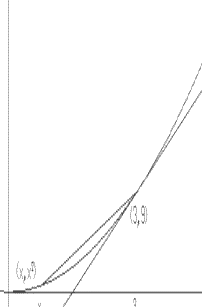Comment: At this stage there are two ways to think of the use of the number represented by "x." First, consider a number, x, as close to (but not equal to) 3 as you can imagine. For this x, the slope of the secant line is $\bar{m}(x)= x+3$, which is very close to 6. We'll describe this way of thinking of the estimation as a static viewpoint, because the number x is considered fixed.
A second way to think of this situation is that we are progressively choosing values for x closer and closer to (but never equal to) 3. For these x's, the slopes of the corresponding secant lines, $\bar{m}(x)$, which could be computed individually using the expression x + 3, are closer and closer to the number 6. We'll describe this way of thinking of the estimation as a dynamic viewpoint because the number x is considered changing, varying close to 3.
Notation: There are some important notation conventions and linguistic expressions that have developed over hundreds of years to represent the kind of analysis just made. We discuss now two ways that will be used throughout the text and are common in mathematics today.

First, it is common to write $x \approx 3$ to represent a statement that x is close (is approximately equal) to 3. In using this notation we also will allow x to equal 3 and will state explicitly when we exclude that possibility. With this notation we can express the static viewpoint of our analysis. We supposed $x \approx 3$ with $x \ne 3$ and found the slope of the corresponding secant line, $\bar{m}(x) \approx 6$.

A second very important notation represents either the static or dynamic viewpoint of estimation with an arrow. With this notation we write $x \rightarrow 3$ for the statement that x is close to 3, or that we are considering x taken closer and closer to 3, or that  x approaches 3. It is usually presumed that $x \ne 3$, but at times it is convenient to allow this notation to include the possibility that the number x may equal 3.
We can express either the static or the dynamic viewpoint of our tangent line analysis with this notation. We supposed that $x \rightarrow 3$ with $x \ne 3$ and algebraically simplified the slope(s) of the corresponding secant line(s), making it easier to see that the slope(s), $\bar{m}(x) \rightarrow 6$.

 Include a picture of the letter $\bar{m}(x)$  in a car approaching a wall labelled 6,  with the letter $x$  on a clock hand approaching the number 3.

Review of Analysis: We have found a method for approximating the slope of the desired tangent line. We analyzed the slopes of secant lines, $\bar{m}(x)$, which were controlled by the choice of numbers for x, the first coordinate of another point on the graph of $Y=X^2$. When $x \rightarrow 3$, with $x \ne 3$, by simplifying algebraically we found that $\bar{m}(x) \rightarrow 6$. Since when $x \rightarrow 3$ the slopes of secant lines are better estimates for the slope of the tangent line, we concluded the slope of the tangent line must be 6.

 Generalization: Frequently a single example has enough of the basic idea of a solution to a problem that it can be used as a template to organize a more general analysis, either as a computation or an argument. When the example has dealt with a specific number, say 3, and the more general analysis replaces that specific number with a  general number represented symbolically, for instance using the letter a, we say we have generalized the example from the specific number, 3, to any real number, a.
Example I.A.2: Generalization for
$Y = X^2$. We continue our estimation analysis to find the slope of the line tangent to the graph of $Y = X^2$ at a generic point with coordinates $(a, a^2)$. We consider the slope of a secant line formed by this point and the point $(x,x^2)$ which we  again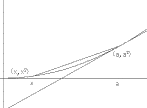denote $\bar m (x)$. See Figure 5.

Using algebra we simplify (since x is not equal to a) to find $\bar{m}(x)= \frac {x^2-a^2}{x-a} = \frac {(x+a)(x-a)}{x-a} = x+a$.
When  $x \rightarrow a, x \ne a$, it should make sense now that  $x + a \rightarrow 2a$ and thus $\bar {m}(x) \rightarrow 2a$.
Therefore, since when $x \rightarrow a$ the slopes of the secant lines improve as estimates for the slope of the tangent line, the slope of the tangent line at the point with coordinates $(a, a^2)$ must be 2a.

Comment: The technique of estimation has become one of the most effective tools for organizing the study of the tangent problem. It is not clear when the precise exposition of the method was first made, but it is certain that the concepts used in this technique were being developed by many individuals prior to the work of Newton and Leibniz. Perhaps it was the blending of these ideas with algebra that began to yield results in a more systematic fashion, raising expectations in those days of achieving a more systematic solution to many other problems as well.
The next few examples illustrate some of the power and ease that the interaction of algebra with the analysis of estimation brings to solving tangent problems.

Example I.A.3. Find an equation for the line tangent to the graph of the equation $Y = 3X^2 - X + 1$ at the point $(1,3)$.
Solution: We find the slope of the required line using the estimation method of the last examples. Let $\bar{m}(x)$ denote the slope of a secant line for the curve that passes through the point $(1, 3)$ and the point on the graph with coordinates $(x, 3x^2 - x + 1)$. Simple algebra now shows that when $x \ne 1$, $\bar{m}(x) =\frac {(3x^2 -x +1)-3}{x-1} =\frac {(3x+2)(x-1)}{x-1}=3x+2$. Thus when $x \rightarrow 1$ with $x \ne 1$, $\bar{m}(x)=3x+2 \rightarrow 5$, so the slope of the tangent line must be 5. Using the point slope formula we conclude by finding an equation for the tangent line: $Y = 5 (X - 1) + 3$.

Example I.A.4. Find the slope of the line tangent to the graph of  $Y = X^3$ at the point $(a,a^3)$.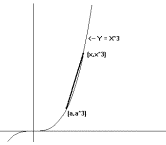Solution: We find the slope of the required line again using the estimation method. Let $\bar{m}(x)$ denote the slope of a secant line for the curve that passes through the point $(a,a^3)$ and the point $(x, x^3)$. [See Figure 1.7.] Simple algebra now shows that when $x \ne a$, $\bar{m}(x) = \frac{x^3-a^3}{x-a} = \frac {(x^2+ax+a^2)(x-a)}{x-a}=x^2 +ax+a^2$  Thus when $x \rightarrow a$ with $x \ne a$, $\bar{m}(x)= x^2+ax+a^2 \rightarrow 3a^2$, so the slope of the tangent line must be $3a^2$.

Comment:
Notice that the slope in this last example is a non-negative number for any a. Based on the sketch of the graph of $Y=X^3$, can you explain why this is so?

Example I.A.5. Find the slope of the line tangent to the graph of $Y=\frac 1X$ at the point $(a, \frac 1a)$ where $a \ne 0$.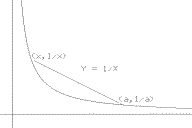Solution: Again we use the method of estimation of the last examples. Let $\bar{m}(x)$ denote the slope of a secant line for the curve that passes through the point $(a,\frac 1a)$ and the point $(x,\frac 1x)$. [See Figure 8.] Simple algebra now shows  that when $x \ne a$ and $x$ and $a \ne 0$, $\bar{m}(x) = \frac{\frac 1x - \frac 1a}{x-a}= \frac{\frac {a-x}{xa}}{x-a}= \frac{a-x}{x-a} \frac 1 {xa} = -\frac1{xa}$.
Thus when $x \rightarrow a$ and $x$ and $a \ne 0$, $\bar{m}(x) = -\frac 1{xa} \rightarrow -\frac 1{a^2}$, so the slope of the tangent line must be $-\frac 1{a^2}$.

Comments: Notice that the slope in this last example is always a  negative number  for any $a$. Based on the sketch of the graph of $Y = \frac 1X$, can you explain why this is so?

Compare the last two examples. Describe the relationship of the sign of the slope of the tangent line and the graph of the equation?

Tool for Estimating Tangent Line Slopes and Instantaneous Velocity

Exercises I.A. For the equations in problems 1 through 7, use estimation analysis to find the slope and an equation of the line tangent to the graph of the equation at the indicated point.
1. $Y = X^2 - X$ ;     a) (1,0)            b) (-1,2)          c) $(a, a^2 -a)$.
2. $Y = 2X^2 - 3X + 1$ ;      a) (1,0)            b) (-1, 6)         c) $(s, 2s^2 - 3s +1)$.
3. $Y = X^3 - X$;                  a) (1,0)            b) (-1,0)          c) $(a, a^3 - a)$.
4. $Y = X^3 - X^2$;   a) (1,0)            b) (-1, -2)        c) $(t, t^3 - t^2)$.
5. $Y = X^4$;              a) (1,1)            b) (2,16)         c) $(a,a^4)$.
6. $Y = \frac 1{X^2}$,   $X \ne 0$ ;      a) (2,.25)        b) $(a, \frac 1{a^2})$.
7. $Y=\sqrt X$, $X \gt 0$ ;     a) (9,3)            b) $(a,\sqrt a)$.

8. For each of the following, use your calculator to estimate the slope of the line tangent to the graph of this equation at (0,0) with secant lines when $x = 0.2, 0.1, 0.05, -0.2, -0.1,$ and $-.05$. Discuss briefly what you think the slope of the tangent might be.        a) $Y = \sin(X)$.                        b) $Y = \tan(X)$.

9. Use a graphing utility to sketch graphs of $Y = X^2$ with the secant lines determined by the point (3,9) with $x = 2.9, 2.99, 2.999, 3.1, 3.01$, and $3.001$. Each of these should use a different viewing window to allow the two points to be visible easily. Comment on the relation of the curve to the secant lines.

10. For each of the following equations use your calculator to estimate the slope of the tangent line at $(0,1)$ using a secant line when $x=0.001$.  a) $Y = 2^x$        b) $Y = 3^x$        c)$Y = (0.5)^x$     d) $Y = (\frac 13)^x$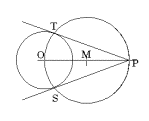Tangents constructed from given points not on a curve
. One of the traditional problems for tangents and circles is to construct tangent lines to a circle through a point given outside of the circle. See Figure 7. The construction from the point P to the circle with center O finds the midpoint M of the segment $\bar{OP}$ and then constructs the circle with radius $\bar{OM}$ and center $M$. The points where the two circles meet are $T$ and $S$, and the lines $TM$ and $SM$ are both tangent to the circle.

The next problems ask you to solve comparable problems for some other curves.

11.Use the result of Example I.A.2 to find equations for any lines that are tangent to the graph of $Y = X^2$ and pass through the point $(3,5)$.
12. Use the result of Example I.A.4 find equations for any lines that are tangent to the graph of $Y = X^3$ and pass through the point $(2,1)$.
13. Use the result of Example I.A.5  to find equations for any lines that are tangent to the graph of $Y =\frac 1X$ and pass through a) $(1,\frac 34)$ ;       b) $(1,\frac12)$.
14. Find the X-intercept of the line that is tangent to the graph of $Y = X^2 - 2$ and passes through the point $(2,2)$.

Normals to Curves: The following exercises explore some properties of normal lines.
15. Find the equation for the normal line to the parabola $Y=X^2$ at $(a, a^2)$.
16. Find the equation for the normal line to the hyperbola $Y=\frac 1X$ at the point $(a, \frac 1a)$, $a \ne 0$.

17. Tangents and the vertex of a parabola.
Consider the parabolic graph of the function $y=f(x) = x^2- 4x +2$. Find the slope of the tangent at the point $(a, a^2 -4a +2)$.
Discuss why the slope of the tangent line at the vertex of a parabola determined by a quadratic function is 0. Use this fact to find the vertex of the parabola with equation $y=f(x) = x^2- 4x +2$.
Generalize this example to show the vertex of a parabola determined by the quadratic function $y=f(x) = Ax^2 +Bx +C$ has first coordinate $x=-\frac B{2A}$.

By the time of Archimedes and Apollonius (dates), Greeks geometers were familiar with the properties of parabolas and their tangents. In the following exercises we investigate one of these relations known to the Greeks and discussed in our preliminary work on parabolas and tangents in section ***.
18. Use $y=x^2$.
a) Show the slope of the tangent line is $m$ at the point when $x= \frac m2$.
b) Find slope of secant line between $(a,a^2)$ and $(b,b^2)$.

c) Show that the tangent line to $y=x^2$
at the midpoint between $x=a$ and $x=b$ is parallel to the secant line between $(a,a^2)$ and $(b,b^2)$.
19. Use $y=x^2 + 6x$.
a) Show the slope of the tangent line is $m$ at the point $x=\frac {m-6}2$.
b) Find slope of secant line between $(a,a^2 +6a)$ and $(b,b^2+6b)$.
c) Show that the tangent line
to $y=x^2 +6x$ at the midpoint between $a$ and $b$ is parallel to the secant line between $(a,a^2 +6a)$ and $(b,b^2+6b)$.
20. Use $y=Ax^2+Bx+C$.
a) Show the slope of the tangent line is $m$ at the point $x=\frac {m-B}{2A}$.
b) Find slope of secant line between $(a,Aa^2+Ba+C)$ and $(b,Ab^2+Bb+C)$.

c) Show that the tangent line to $y=Ax^2+Bx+C$
at the midpoint between a and b is parallel to the secant line between $(a,Aa^2+Ba+C)$ and $(b,Ab^2+Bb+C)$.
21. Using symmetrically placed points to estimate the slope of a tangent line: with $y=\frac 1x$ and $y=x^3$.
22. Consider $\frac{\sin(a+h) -\sin(a-h)}{2h}=\frac {\sin(h)\cos(a)}h$ . Estimate the slope of tangent for $y=\sin(x)$ at $(a,\sin(a))$ when $h \rightarrow 0$?
23. On the graph below (Figure 9), estimate the slope of the tangent lines to the given curve at the indicated points.24. Suppose $x \rightarrow 3$, for each of the following expressions, give a number that the expression approaches. Give some numerical evidence for your conclusion.
a) $x^2-3x+2$.
b) $\frac {x^2-9}{x-3}$.
c)
$\frac {x^3-27}{x-3}$.
d)
$\frac {x^2-2x-3}{x-3}$.

25. Seeing the tangent line meet a curve. With the power of graphing technology it is possible to look much more closely at the graph of a function than anyone might have anticipated one hundred years ago. Ability to zoom in on a point until the curve is indistinguishable from a line can allow the viewer to estimate the slope of the tangent line by measuring the slope of the apparent line in the viewing window of a graphical image.
Here are some samples from sections of the graph of some functions with the size of the viewing window. Based on this information, estimate the slope of the tangent line for each of these functions at the indicated point on the graph.